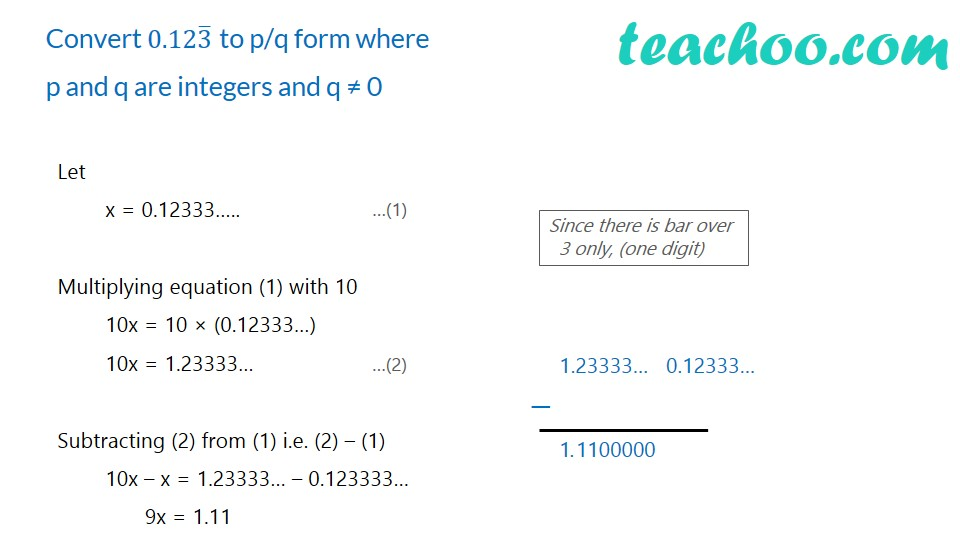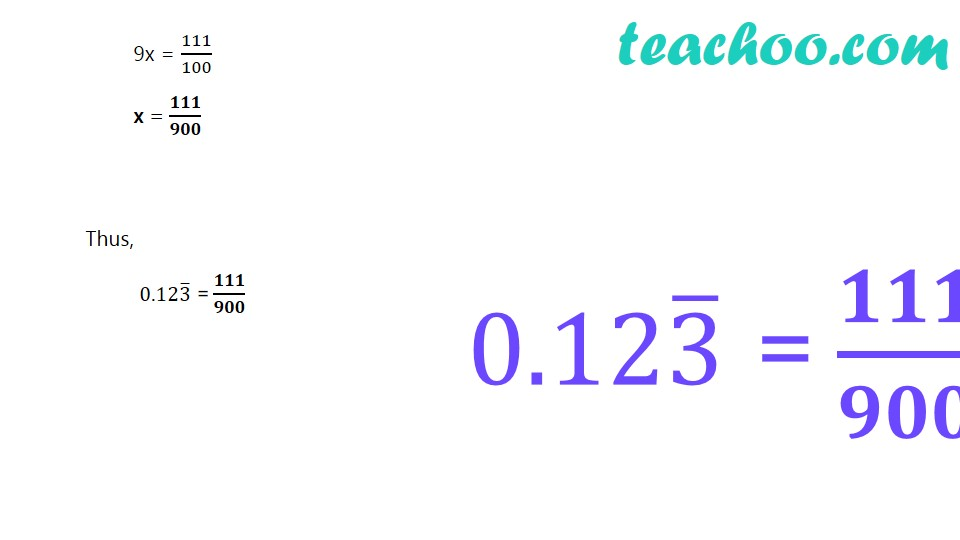Expressing decimal in p/q

Chapter 1 Class 9 Number Systems
Concept wiseLearn in your speed, with individual attention - Teachoo Maths 1-on-1 Class

### Transcript

Convert 0.123 ̅ to p/q form where p and q are integers and q ≠ 0 Let x = 0.12333….. Multiplying equation (1) with 10 10x = 10 × (0.12333…) 10x = 1.23333… Subtracting (2) from (1) i.e. (2) – (1) 10x – x = 1.23333… – 0.123333… 9x = 1.11 Since there is bar over 3 only, (one digit) 1.23333… 0.12333… 9x = 111/100 x = 𝟏𝟏𝟏/𝟗𝟎𝟎 Thus, 0.123 ̅ = 𝟏𝟏𝟏/𝟗𝟎𝟎 0.123 ̅ = 𝟏𝟏𝟏/𝟗𝟎𝟎